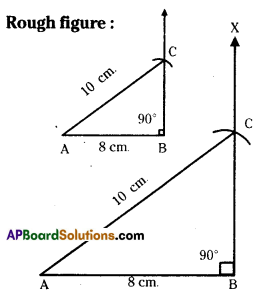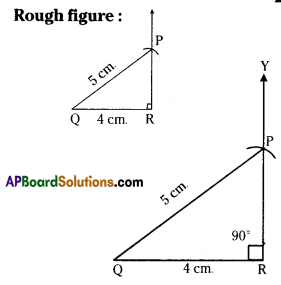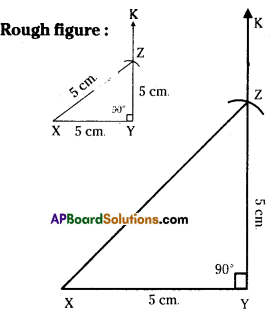# HSSlive: Plus One & Plus Two Notes & Solutions for Kerala State Board

## AP Board Class 7 Maths Chapter 9 Construction of Triangles Ex 4 Textbook Solutions PDF: Download Andhra Pradesh Board STD 7th Maths Chapter 9 Construction of Triangles Ex 4 Book AnswersAP Board Class 7 Maths Chapter 9 Construction of Triangles Ex 4 Textbook Solutions PDF: Download Andhra Pradesh Board STD 7th Maths Chapter 9 Construction of Triangles Ex 4 Book Answers

## Andhra Pradesh State Board Class 7th Maths Chapter 9 Construction of Triangles Ex 4 Books Solutions

 Board AP Board Materials Textbook Solutions/Guide Format DOC/PDF Class 7th Subject Maths Chapters Maths Chapter 9 Construction of Triangles Ex 4 Provider Hsslive

2. Click on the Andhra Pradesh Board Class 7th Maths Chapter 9 Construction of Triangles Ex 4 Answers.
3. Look for your Andhra Pradesh Board STD 7th Maths Chapter 9 Construction of Triangles Ex 4 Textbooks PDF.
4. Now download or read the Andhra Pradesh Board Class 7th Maths Chapter 9 Construction of Triangles Ex 4 Textbook Solutions for PDF Free.

## AP Board Class 7th Maths Chapter 9 Construction of Triangles Ex 4 Textbooks Solutions with Answer PDF Download

Find below the list of all AP Board Class 7th Maths Chapter 9 Construction of Triangles Ex 4 Textbook Solutions for PDF’s for you to download and prepare for the upcoming exams:

Question 1.
Construct a right-angled ΔABC such that ∠B = 90°, AB = 8 cm and AC = 10 cm.
Solution:
∠B = 90°, AB = 8cm, AC = 10 cm.Step -1: Draw a rough sketch of the right angled triangle and label it with the given measurements.
Step -2: Draw a line segment AB of length 8 cm.
Step -3: Draw a ray BX−→− perpendicular to AB to B.
Step -4 : Draw an arc from A with radius 10 cm to intersect BX−→− at C.
Step -5: Join A, C to get the required ΔABC.

Question 2.
Construct a ΔPQR, right-angled at R, hypotenuse is 5 cm and one of its adjacent sides is 4cm.
Solution:
∠R=90D,QR=4cm,PQ=5cm.Step -1: Draw a rough sketch of the right angled triangle and label it with the given measurements.
Step -2: Draw a line segment QR of length 4cm.
Step -3: Draw a ray RY−→− perpendicular to QR at R.
Step -4: Draw an arc from Q with radius 5 cm to intersect RY−→− at P.
Step -5: Join P, Q to get the required ΔPQR.

Question 3.
Construct an isosceles right-angled ΔXYZ in which ∠Y = 90° and the two sides are 5 cm each.
Solution:
∠Y = 90°,XY = YZ = 5cm.Step- 1: Draw a rough sketch of a right angled triangle and label it with given measurements.
Step -2: Draw a line segment XY of length 5cm.
Step -3: Draw a ray YK−→− perpendicular to XY at Y.
Step -4: Draw an arc from Y with radius 5 cm to intersect YK−→− at Z.
Step -5: Join Z, X to get the required ΔXYZ.

## Andhra Pradesh Board Class 7th Maths Chapter 9 Construction of Triangles Ex 4 Textbooks for Exam Preparations

Andhra Pradesh Board Class 7th Maths Chapter 9 Construction of Triangles Ex 4 Textbook Solutions can be of great help in your Andhra Pradesh Board Class 7th Maths Chapter 9 Construction of Triangles Ex 4 exam preparation. The AP Board STD 7th Maths Chapter 9 Construction of Triangles Ex 4 Textbooks study material, used with the English medium textbooks, can help you complete the entire Class 7th Maths Chapter 9 Construction of Triangles Ex 4 Books State Board syllabus with maximum efficiency.

## FAQs Regarding Andhra Pradesh Board Class 7th Maths Chapter 9 Construction of Triangles Ex 4 Textbook Solutions

#### Can we get a Andhra Pradesh State Board Book PDF for all Classes?

Yes you can get Andhra Pradesh Board Text Book PDF for all classes using the links provided in the above article.

## Important Terms

Andhra Pradesh Board Class 7th Maths Chapter 9 Construction of Triangles Ex 4, AP Board Class 7th Maths Chapter 9 Construction of Triangles Ex 4 Textbooks, Andhra Pradesh State Board Class 7th Maths Chapter 9 Construction of Triangles Ex 4, Andhra Pradesh State Board Class 7th Maths Chapter 9 Construction of Triangles Ex 4 Textbook solutions, AP Board Class 7th Maths Chapter 9 Construction of Triangles Ex 4 Textbooks Solutions, Andhra Pradesh Board STD 7th Maths Chapter 9 Construction of Triangles Ex 4, AP Board STD 7th Maths Chapter 9 Construction of Triangles Ex 4 Textbooks, Andhra Pradesh State Board STD 7th Maths Chapter 9 Construction of Triangles Ex 4, Andhra Pradesh State Board STD 7th Maths Chapter 9 Construction of Triangles Ex 4 Textbook solutions, AP Board STD 7th Maths Chapter 9 Construction of Triangles Ex 4 Textbooks Solutions,
Share: